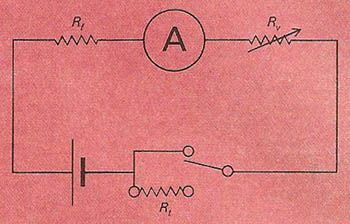A# electrical resistanceSimplified circuit diagram for an ohmmeter. A represents the ammeter, Rf the fixed resistor, Rv the variable resistor, and Rt the resistor under test.

Electrical resistance (R) is the extent to which an electrical conductor hinders the flow of a current. Resistance is the ratio of the potential difference across an electrical component to the current passing through it. It is measured in ohms and is represented by the symbol R. In general, the resistance of a metallic conductor increases with temperature, whereas the resistance of a semiconductor decreases with temperature. In an alternating current, resistance is the real part of the impedance.

Resistance is characteristic of the material of which the conductor is made (the resistance presented by a unit cube of a material being called its resistivity) and of the physical dimensions of the conductor, increasing as the conductor becomes longer and/or thinner. Its accurate measurement is possible using a Wheatstone bridge.

Compare with conductance.

## Resistor

A resistor is a circuit component, with a specified resistance, which is designed to reduce current. A resistor produces a voltage drop between its terminals in accordance with Ohm's law.

Resistors for electronic circuits are generally made from finely ground particles of carbon mixed with a ceramic material and enclosed in an insulated tube. The value of the resistance is given by a coded set of colored rings on the outside of the tube. resistors for carrying larger currents consist of coils of insulated wire.

## Ohmmeter

An ohmmeter is an instrument for providing a rapid, if approximate, value for the resistance of part of an electric circuit (see diagram). It consists of an ammeter (reverse calibrated in ohms in series with a fixed resistance, a battery, and a variable resistor. This last is adjusted to zero the meter with the terminals of the instrument shorted. The test resistance is then introduced between these terminals. The reduction in the current so caused is a measure of the resistance.